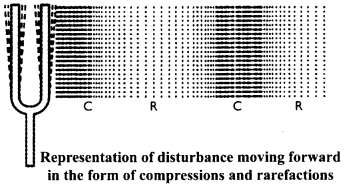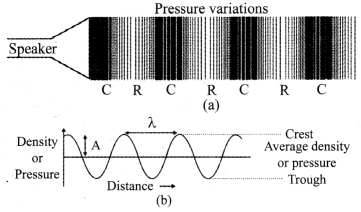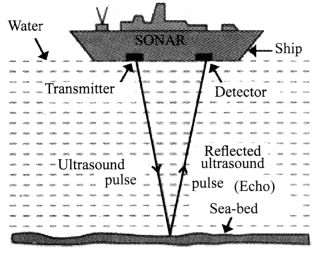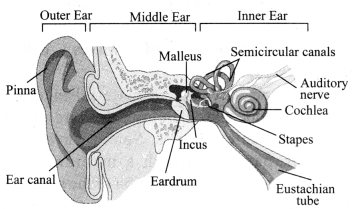# JAC Class 9 Science Notes Chapter 12 Sound

### JAC Board Class 9th Science Chapter 12 Notes Sound

→ Sound: Sound is a form of energy which produces a sensation of hearing in our ears.

→ Production of Sound: Sound is produced due to the vibration of objects. Vibration is a periodic back-and-forth motion of the particles of an elastic body or medium about a central position. It is also called as oscillation.
For example:
a. Strings of a guitar vibrate to produce sound when stretched.
b. When membrane of a table is struck, it vibrates to produce sound.→ Propagation of Sound
a. The travelling of sound is called propagation of sound. Sound is propagated by the to and fro motion of particles of the medium.

b. When an object vibrates, the particles in the medium vibrate. The particle in contact with the vibrating object is first displaced from its equilibrium position. Each particle disturbs the other particle in contact. Thus, the disturbance is carried from the source to the listener.c. Only the disturbance produced by the vibrating body travels through the medium, but the particles do not move forward themselves. Sound waves cannot travel in vacuum.

→ Medium: The matter or substance through which sound is transmitted is called a medium. It can be solid, liquid or gas. Air is the most common medium for sound propagation.

→ Wave: Wave is a disturbance in which energy is transferred from one point to another without any direct contact between them.
For example: heat, light and sound are considered as waves.

→ Types of Waves
On the basis of direction of propagation, waves are categorised into two types:
a. Longitudinal waves: These are the waves in which the particles of the medium vibrate along the direction of propagation of the wave. For example: sound wave.
b. Transverse waves: In this type of wave, the particles of the medium vibrate in a direction perpendicular to the direction of propagation of the wave. For example: waves produced in a stretched string.

Another type of waves which do not require any medium for propagation are called electromagnetic waves. These waves can travel through vacuum. For example, light waves and X-rays.

→ Compressions and Rarefactions

1. Compression is the part of wave in which particles of the medium are close to one another forming a region of high pressure and density. Compressions are represented by the upper portion of the curve called crest.
2. Rarefaction is the part of wave where particles spread out to form a region of low pressure and density. Rarefactions are represented by the lower portion of the curve called trough.→ Wavelength: Distance between two consecutive crests or troughs is called wavelength. Wavelength is represented by λ (lambda) and its SI unit is metre.

→ Frequency: Number of oscillations of a sound wave per unit time is called its frequency. The number of crests or troughs per unit of time will give the frequency. Its SI unit is hertz (Hz).

→ Time period: The time taken to complete one vibration is called time period. It is denoted by ‘T’. Its SI unit is second (s). The frequency of a wave is the reciprocal of the time period.
v = $$\frac{1}{\mathrm{~T}}$$; where v is the frequency and T is the time period.

→ Amplitude: The magnitude ofthe maximum disturbance in the medium, on either side of the mean value, is called the amplitude of the wave. It is usually represented by the letter ‘A’. Its unit is metre.→ Velocity: The distance travelled by a wave in one second is called velocity of the wave or speed of the wave. Its SI unit is metre per second (m s-1).
Velocity = $$\frac{\text { Distance travelled }}{\text { Time taken }}$$
v = $$\frac{\lambda}{\mathrm{T}}$$, where λ = wavelength of the wave travelled and T is the time period.
v = λv (As $$\frac{1}{\mathrm{~T}}$$ = v, where v is called frequency of the wave)
Thus, Velocity = Wavelength × Frequency
v = λv is called the wave equation.

→ Pitch and Loudness of Sound
a. Pitch: It represents shrillness or flatness of sound. It depends on the frequency of vibration. The higher the frequency of sound wave, the higher will be the pitch of sound and vice-versa.
b. Loudness: It is the measure of the sound energy reaching the ear per second. It depends on the amplitude of the sound wave. It is measured in decibel ‘dB’.

→ Music and Noise
a. Music: It is the sound that is pleasant to hear. For example, sound coming from the musical instruments.
b. Noise: It is the sound that is unpleasant to hear. For example, sound produced by vehicles.

→ Tone and Note
a. Tone: A pure sound of single frequency is called tone.
b. Note: An impure sound produced by a mixture of many frequencies is called a note. For example, a musical note has tones of various frequencies.

→ Audible Frequency: The audible range of human ear is 20 Hz to 20,000 Hz, i.e., human ear can hear only those waves whose frequencies fall between 20 Hz and 20,000 Hz.

→ Infrasonic sound: Sound whose frequency is less than 20 Hz is called infrasonic sound. Ultrasonic sound: Sound whose frequency is more than 20,000 Hz is called ultrasonic sound.

→ Uses of ultrasonic sound: Ultrasonic sound is used to clean objects like electronic components, to detect cracks in metal blocks, in ultrasound scanners for getting images of internal organs of the human body, to break small stones formed in the kidneys into fine grains, etc.

→ Reflection of Sound: Sound reflects off a surface in the same way as light reflects and follows the same rules of reflection. Hard surfaces reflect sound better than the soft ones.→ Laws of reflection of sound

• The directions in which the sound is incident and reflected make equal angles with the normal to the reflecting surface at the point of incidence.
• The incident sound wave, the reflected sound wave and the normal all, lie in the same plane.
• The reflection of sound has many applications such as stethoscopes, horns, megaphones, designing of concert halls, etc.

→ Echo: In bigger monuments and in large empty halls, you can hear reflections of your sound after you speak something. This effect is known as echo. For hearing a distinct echo, the time interval between the original sound and the reflected one must be at least 0.1 s.

Since the speed of sound in air is 344 m/s, the distance travelled by sound in 0.1 s is 34.4 m. So, to hear an echo clearly and distinctly, the minimum distance of the reflecting surface from the source of sound should be half of this distance, i.e., 17.2 m.

→ Reverberation: The persistence of sound in an auditorium as a result of repeated reflections of sound is called reverberation.

→ Sonar: It is a device which uses ultrasonic waves to measure the distance, direction and speed of underwater objects. The distance of the object can be calculated by knowing the speed of sound in water and the time taken between the transmission and reception of ultrasound.→ Structure of the human ear: The sound waves pass through the ear canal onto a thin membrane called eardrum. The eardrum vibrates and the vibrations are amplified by the three bones of the middle ear called malleus, incus and stapes. Middle ear then transmits the sound waves to the inner ear. The brain then interprets the signals as sound.→ Supersonic speed: When a body moves in air with a speed greater than the speed of sound, it is said to have a supersonic speed.

→ Shock wave: When a supersonic aircraft moves through air, it leaves behind itself a conical region of disturbance which spreads continuously. Such a disturbance is called a shock wave. Shock waves travel at supersonic speeds and carry a huge amount of energy.